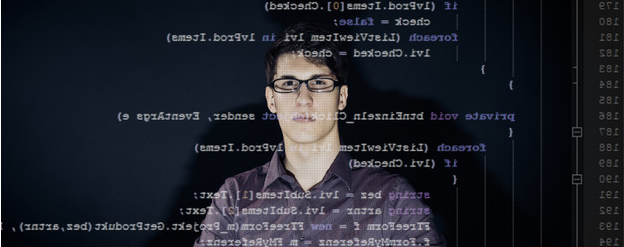# 教你4种方法提高Excel中VBA的效率

##一、尽量使用VBA原有的属性、方法和Worksheet函数

For Each c In
Worksheet(1).Range(″A1:A1000″)
TotalValue = TotalValue ＋ c.Value
Next

AverageValue = TotalValue / Worksheet(1).Range(″A1:A1000″).Rows.Count

AverageValue=Application.WorksheetFunction.Average(Worksheets(1).Range( ″

A1:A1000″))

## 二、尽量减少使用对象引用，尤其在循环中

1．使用With语句
Workbooks(1).Sheets(1).Range(″A1:A1000″).Font.Name=″Pay″

Workbooks(1).Sheets(1).Range(″A1:A1000″).Font.FontStyle=″Bold″ ...

With Workbooks(1).Sheets(1).Range(″A1:A1000″).Font
.Name = ″Pay″
.FontStyle = ″Bold″
...

End With

2．使用对象变量

Workbooks(1).Sheets(1).Range(″A1″).Value = 100

Workbooks(1).Sheets(1).Range(″A2″).Value = 200

Set MySheet = Workbooks(1).Sheets(1)
MySheet.Range(″A1″).Value = 100

MySheet.Range(″A2″).Value = 200

3．在循环中要尽量减少对象的访问

For k = 1 To 1000
Sheets(″Sheet1″).Select
Cells(k,1).Value = Cells(1,1).Value
Next k

Set TheValue = Cells(1,1).Value
Sheets(″Sheet1″).Select
For k = 1 To 1000
Cells(k,1).Value = TheValue

Next k## 三、减少对象的激活和选择

Sheets(″Sheet3″).Select
Range(″A1″).Value = 100
Range(″A2″).Value = 200

With Sheets(″Sheet3″)
.Range(″A1″).Value = 100
.Range(″A2″).Value = 200

End With

## 四、关闭屏幕更新

Application.ScreenUpdate = False

Application.ScreenUpdate = True

App下载